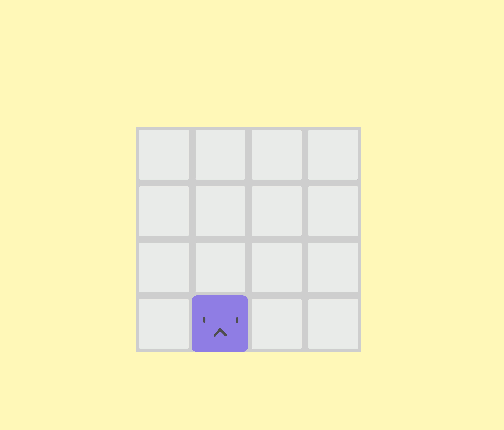# Why is my vertical movement faster than my horizontal?My PlayerController.cs code:

``````using UnityEngine;

[RequireComponent(typeof(Rigidbody2D))]
public class PlayerController : MonoBehaviour
{
Rigidbody2D rb2d;
bool isMoving;

public float moveSpeed = 500f;

private void Start()
{
rb2d = this.gameObject.GetComponent<Rigidbody2D>();
}

public void Move()
{
float moveX = 0;
float moveY = 0;

// Only let player moves when they are not moving
if (isMoving == false)
{
moveX = Input.GetAxisRaw("Horizontal");
moveY = Input.GetAxisRaw("Vertical");
}

if (moveX != 0 && moveY != 0)
{
// If player tries to move diagonally, return nothing
return;
}

if (moveX != 0 || moveY != 0)
{
Vector2 moveVelocity = new Vector2(moveX, moveY).normalized * (Time.deltaTime * moveSpeed);
rb2d.velocity += moveVelocity;
Debug.Log(rb2d.velocity);
isMoving = true;
}

if (rb2d.velocity.x == 0 && rb2d.velocity.x == 0)
{
isMoving = false;
}
}
}
``````

change the last if you are only compary the x velocity no the y so when you add the Y velocity isMoving is always false

``````     if (rb2d.velocity.x == 0 && rb2d.velocity.y == 0)
{
isMoving = false;
}
``````